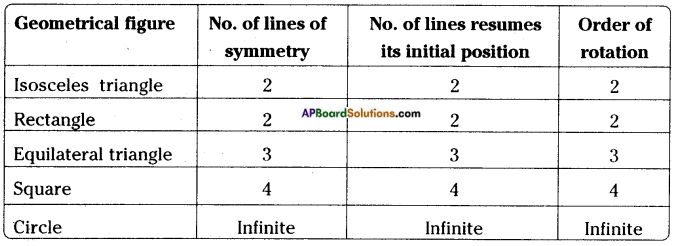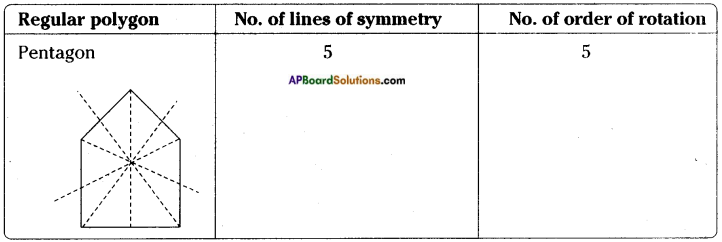## AP State Syllabus 8th Class Maths Solutions 8th Lesson Exploring Geometrical Figures InText Questions

AP State Syllabus AP Board 8th Class Maths Solutions Chapter 8 Exploring Geometrical Figures InText Questions and Answers.

### 8th Class Maths 8th Lesson Exploring Geometrical Figures InText Questions and Answers

Do this

Question 1.
Identify which of the following pairs of figures are congruent.     [Page No. 184]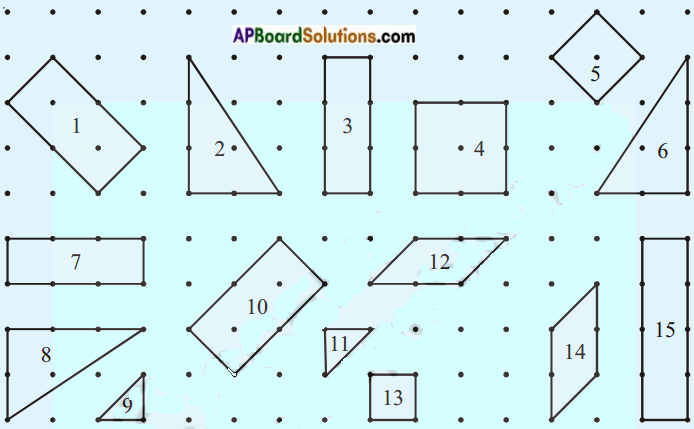The congruent figures are (1, 10), (2, 6, 8), (3, 7), (12, 14), (9, 11), (4, 13).Question 2.
Look at the following pairs of figures and find whether they are congruent. Give reasons. Name them.    [Page No. 185]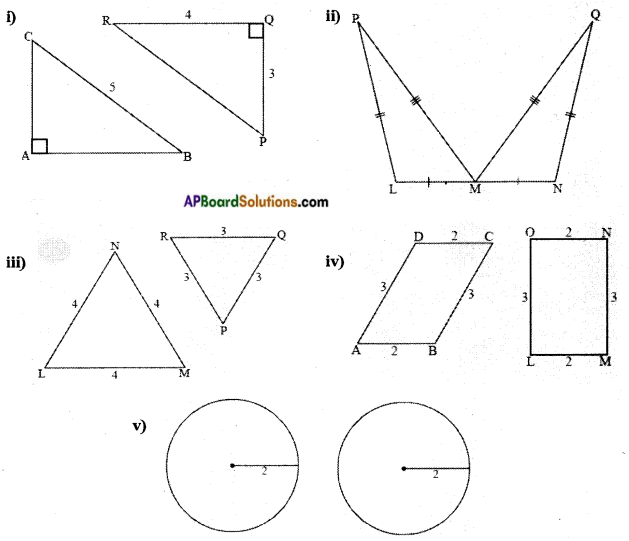i) △ABC, △PQR
∠A = ∠Q Angle
There is no information about other angles (or) sides.
But if we overlap each other, they coincide.
∴ △ABC ≅ △PQR

ii) From △PLM, △QNM
PL = QN (S)
LM = MN (S)
PM = QM (S)
By S.S.S congruency, these two triangles are congruent.
∴ △PLM ≅ △QNM

iii) From △LMN, △PQR
NL ≠ PQ,LM ≠ QR, NM ≠ RP [∵ The corresponding angles are not given]
∴ △LMN ≆ △PQR

iv) From fig. ABCD is a parallelogram and LMNO is a rectangle.
In any case a rectangle and a parallelogram are not congruent.
∴ ▱ ABCD ≆ □ DLMNO

v) Both the circles are having same radii,
i.e., r1 = r2 = 2 units
∴ The given circles are congruent to each other.Question 3.
Identify the out line figures which are similar to those given first.    [Page No. 186]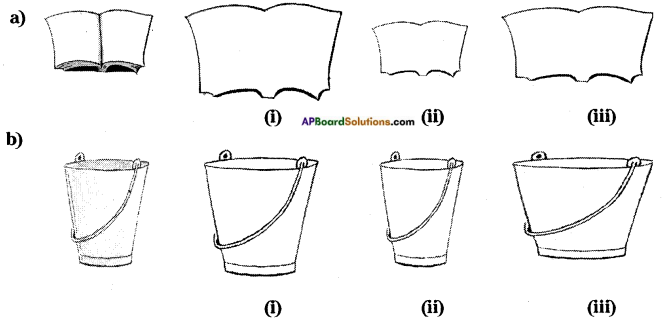The similar figures are (a) (ii), (b) (ii).

Question 4.
Draw a triangle on a graph sheet and draw its dilation with scale factor 3. Are those two figures are similar?      [Page No. 191]
Step – 1: Draw a △ PQR and choose the center of dilation C which is not on the triangle. Join every vertex of the triangle from C and produce.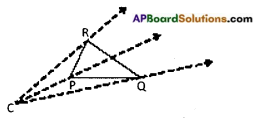Step – 2: By using compasses, mark three points P’, Q’ and R’ on the projections
so that
CP’ = k(CP) = 3CP
CQ’ = 3 CQ
CR’ = 3 CR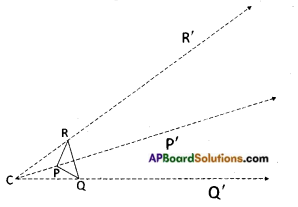Step- 3: Join P’Q’,Q’R’and R’P’.
Notice that △P’Q’R’ ~ △PQR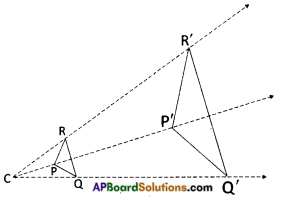Question 5.
Try to extend the projection for any other diagram and draw squares with scale factor 4, 5. What do you observe? [Page No. 191]
Sometimes we need to enlarge 10 the figures say for example while making cutouts, and sometimes we reduce the figures during designing. Here in every case the figures must be similar to the original. This means we need to draw enlarged or reduced similar figures in daily life. This method of drawing enlarged or reduced similar figure is called ‘Dilation’.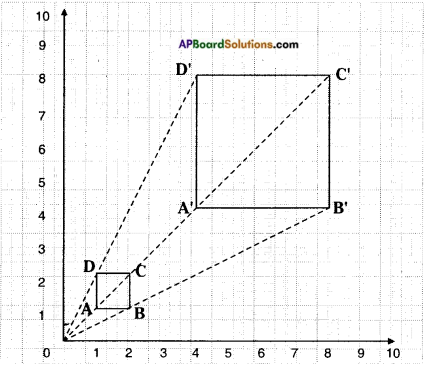Observe the following dilation ABCD, it is a square drawn on a graph sheet.
Every vertex A, B, C, D are joined from the sign ‘O’ and produced to 4 times the length upto A, B, C and D respectively. Then A, B, C, Dare joined to form a square which 4 times has enlarged sides of ABCD. Here, 0 is called centre of dilation and
$$\frac{OA’}{OA}$$ = $$\frac{4}{1}$$ = 4 is called scale factor.

Question 6.
Draw all possible lines of symmetry for the following figures.     [Page No. 193]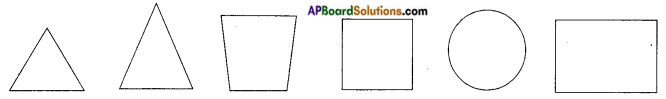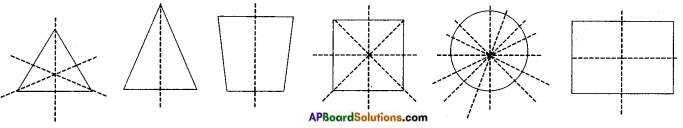Try theseQuestion 1.
Stretch your hand, holding a scale in your hand vertically and try to cover your school building by the scale (Adjust your distance from the building). Draw the figure and estimate height of the school building.      [Page No. 189]
Illustration: A girl stretched her arm towards a school building, holding a scale vertically in her arm by standing at a certain distance from the school building. She found that the scale exactly covers the school building as in figure. If we compare this illustration with the previous example, we can say that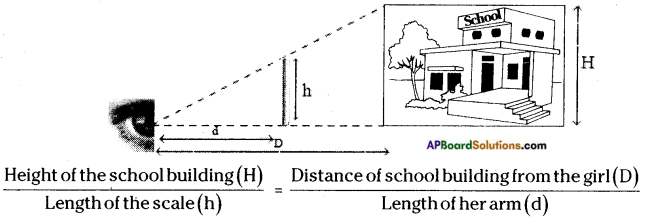By measuring the length of the scale, length of her arm and distance of the school building, we can estimate the height of the school building.

Question 2.
Identify which of the following have point symmetry.     [Page No. 196]
1.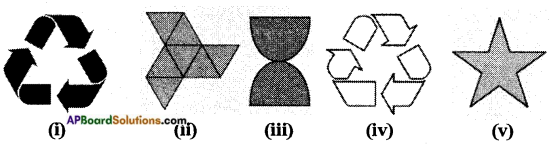2. Which of the above figures are having symmetry ?
3. What can you say about the relation between line symmetry and point symmetry?
1. The figures which have point symmetry are (i), (ii), (iii), (v).
2. (i), (iii), (v).
3. Number of lines of symmetry = Order of point symmetry.

Think, discuss and write

Question 1.
What is the relation between order of rotation and number of axes of symmetry of a geometrical figure?     [Page No. 195]